# Power System Components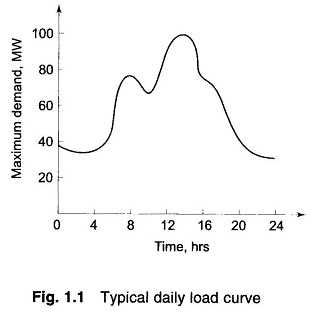What is Load Curve?: Load drawn by consumers is the toughest parameter to assess scientifically. The magnitude of the load, in fact, changes continuously so that the load forecasting problem is truly a statistical one. A typical daily Representation of Load Curve is shown in Fig. 1.1. The loads are generally composed of industrial and …

## Salient Pole Synchronous Generator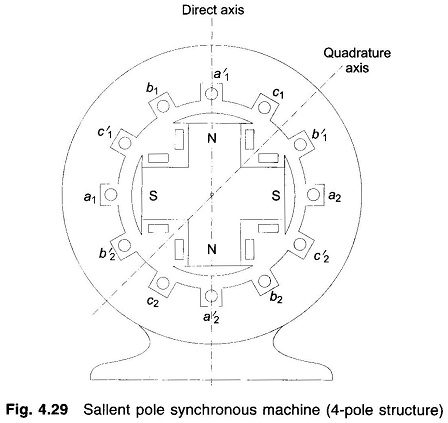Salient Pole Synchronous Generator: A Salient Pole Synchronous Generator, as shown in Fig. 4.29, is distinguished from a round rotor machine by constructional features of field poles which project with a large interpolar air gap. This type of construction is commonly employed in machines coupled to hydroelectric turbines which are inherently slow-speed ones so that …

## Phasor Diagram of Synchronous Motor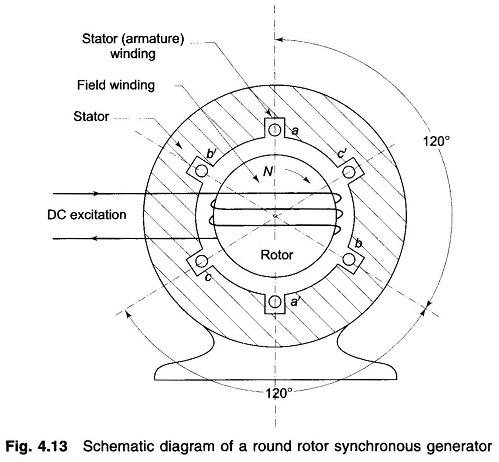Phasor Diagram of Synchronous Motor: The Phasor Diagram of Synchronous Motor is the most important element of a power system. It converts mechanical power into electrical form and feeds it into the power network or, in the case of a motor, it draws electrical power from the network and converts it into the mechanical form. …

## Per Unit System in Power System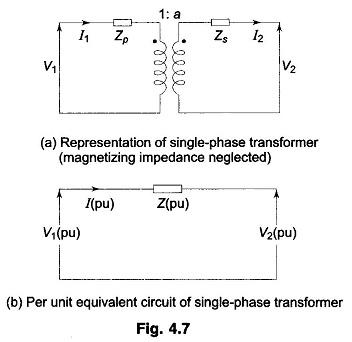Per Unit System in Power System: Per Unit System in power system is usual to express voltage, current, voltamperes and impedance of an electrical circuit in per unit (or percentage) of base or reference values of these quantities. The per unit value of any quantity is defined as: The Per Unit System in Power System …

## Single Line Diagram of Power System and Impedance or Reactance Diagram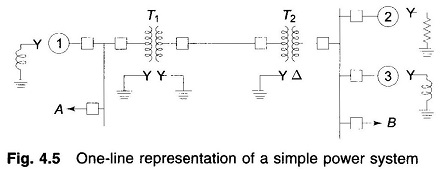Single Line Diagram of Power System and Impedance or Reactance Diagram: A single line diagram of power system shows the main connections and arrangements of components. Any particular component may or may not be shown depending on the information required in a system study, e.g. circuit breakers need not be shown in a load flow …

## Balanced Three Phase Circuit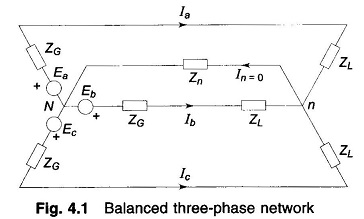Balanced Three Phase Circuit: The solution of a balanced three phase circuit is easily carried out by solving the single-phase network corresponding to the reference phase. Figure 4.1 shows a simple, balanced three phase circuit. The generator and load neutrals are therefore at the same potential, so that In = 0. Thus the neutral impedance …

Scroll to Top